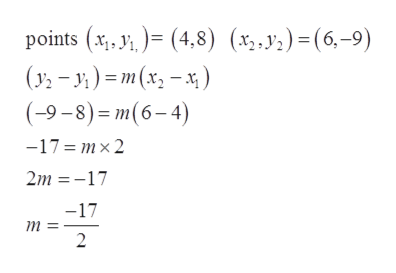# What would be the linear equation for this function, & the graph??(X1,Y1)=(4,8), (X2,Y2)=(6,-9)

Question
1 views

What would be the linear equation for this function, & the graph??

(X1,Y1)=(4,8), (X2,Y2)=(6,-9)

check_circle

Step 1

Given:

Step 2

Formula used:

Step 3

So, by the Point-Slope form of line the ...help_outlineImage Transcriptioncloseрoints (x, 3,)- (4,8) (у» - у) -т(x, - *) (-9-8)- т(6-4) -17%3 тx2 (х.у.) - (6,-9) 2m 17 -17 т 2 fullscreen

### Want to see the full answer?

See Solution

#### Want to see this answer and more?

Solutions are written by subject experts who are available 24/7. Questions are typically answered within 1 hour.*

See Solution
*Response times may vary by subject and question.
Tagged in

### Other